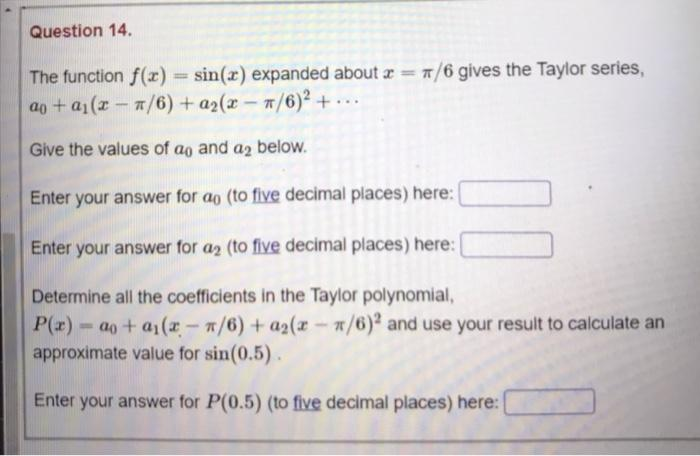### Create an Account

Already have account?

### Forgot Your Password ?

Home / Questions / Question 14. The function f(x) = sin(x) expanded about x = 7/6 gives the Taylor series, aj...

# Question 14. The function f(x) = sin(x) expanded about x = 7/6 gives the Taylor series, aj + a1(x - 7/6) + a2(x - #/6)+ ... Give the values of an and a2 below. Enter your answer for ao (to five decima

Question 14. The function f(x) = sin(x) expanded about x = 7/6 gives the Taylor series, aj + a1(x - 7/6) + a2(x - #/6)+ ... Give the values of an and a2 below. Enter your answer for ao (to five decimal places) here: II Enter your answer for az (to five decimal places) here: Determine all the coefficients in the Taylor polynomial, P(x) - 20+ 01(x - #/6) + a2(x - 7/6) and use your result to calculate an approximate value for sin(0.5) Enter your answer for P(0.5) (to five decimal places) here:Apr 15 2021 View more View Less

#### Answer (Solved)Subscribe To Get Solution